# Factorisation of Polynomials and Algebraic Identities Class 9 Notes | EduRev

## Class 9 : Factorisation of Polynomials and Algebraic Identities Class 9 Notes | EduRev

The document Factorisation of Polynomials and Algebraic Identities Class 9 Notes | EduRev is a part of the Class 9 Course Mathematics (Maths) Class 9.
All you need of Class 9 at this link: Class 9

Factor Theorem : If p(x) is a polynomial of degree n > 1 and a is any real number,
then
(i) x – a is a factor of p(x), if p(a) = 0, and
(ii) p(a) = 0, if x – a is a factor of p(x).

Proof: By the Remainder Theorem, p(x)=(x – a) q(x) + p(a).
(i) If p(a) = 0, then p(x) = (x – a) q(x), which shows that x – a is a factor of p(x).
(ii) Since x – a is a factor of p(x), p(x) = (x – a) g(x) for same polynomial g(x).
In this case, p(a) = (a – a) g(a) = 0.

Example 1:
Factor pq - pr - 3ps
Solution:
= pq - pr - 3ps
To factor the above algebraic expression,first we have ask a question for ourself

Question : Do we find any common term in the above algebraic expression ?
Yes, we have " p" in all three terms.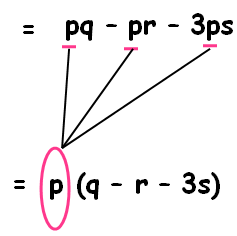So the answer is p (q - r- 3s)
Now we are going to see the next example of the topic"factoring polynomials".

Example 2:
Factor 4a - 8b + 5ax - 10bx
Solution:
4a - 8b + 5ax - 10bx
We have 4 terms in the given algebraic expression.Now we are going to split into two groups.
4a and -8b are in one group (Group 1)
5ax and -10bx are in the other group(Group 2)

Question : Do we have any common variable in group 1?

Question : Do we have any common variable in group 2?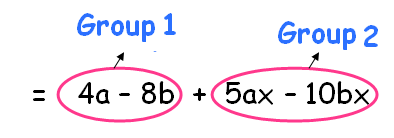Question : Do we have any common number in group 1?
Answer: Yes, we can split 8 as multiple of 4.

Question : Do we have any common number group 2?
Answer: Yes, we can split 10 as multiple of 5.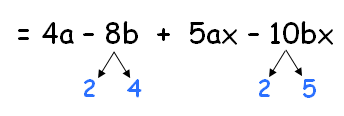Factor out the common term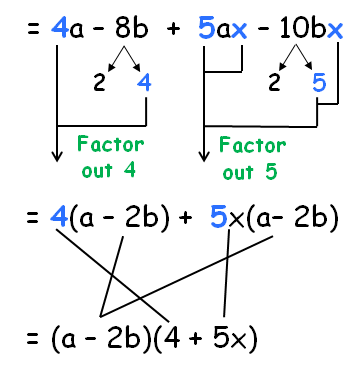So, the factors are (a - 2b) (4 + 5x)
Now we are going to see the next example of the topic"factoring polynomials".

Example 3:
Factor 2a³ - 3a²b + 2a²c
Solution :
= 2a³ - 3a²b + 2a²c

Question : Do we find any common variable or number in all three terms?
Answer: yes,we have a² in all three terms.
= a² (2a - 3b + 2c)
Now we are going to see the next example of the topic"factoring polynomials".

Example 4:
Factor 10x³ - 25x⁴ y
Solution :
10x³ - 25 x⁴ y

Question : Do we find any common variable or number in all three terms?
Answer: yes,we have x³ and for number we can split both 10 and 25 as the multiple of 5.So we have 5x³ as common term.
= 5x³ (2 - 5xy)
Now we are going to see the next example of the topic"factoring polynomials".

How to factor trinomials with 2 different variables using algebraic identities

More examples of factoring polynomials
Example 5:
Factorize 9x² - 24xy + 16y²
Solution:
We have x² as the first term and y² as the last term.Since there are only three terms.We can compare the given question with the algebraic identity a² - 2ab + b²
=  9x² - 24xy + 16y²
=  3² x² - 2(3x) (4y) + 4² y²
=  (3 x)² - 2(3x) (4y) + (4 y)²
=  (3x - 4y)²
Now we are going to see the next example of the topic"factoring polynomials".

Example 6:
Factorize 64 a³ - 343 b³
Solution:
We can  split 64 as 4 x 4 x 4 and we can split 343 as  7 x 7 x 7.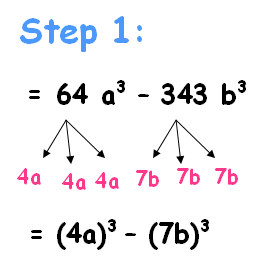a³ - b³ = (a - b) (a² + ab + b²)

by comparing the given polynomial (4a)³ - (7b)³,we get
= (4a - 7b) [(4a)² + (4a) (7b) + (7b)²]
= (4a - 7b) (16a² + 28ab + 49b²)
Now we are going to see the next example of the topic"factoring polynomials".

(i) If the coefficient is 1 we have to take the constant termand we have to split it as two parts.
(ii) The product of two parts must be equal to the constant term and the simplified value
must be equal to the middle term (or) x term.
(iii) Now we have to write these numbers in the form of (x + a) and (x +b)

Factoring quadratic equations when a isn't 1
(i) If it is not 1 then we have to multiply the coefficient of x² by the constant term and we have to split it as two parts.
(ii) The product of two parts must be equal to the constant term and the simplified value must be equal to the middle term (or) x term.
(iii) Divide the factors by the coefficient of x². Simplify the factors by the coefficient of x² as much as possible.
(iv) Write the remaining number along with x.

Let us see some examples for better understanding.
Example 7:
Factor x² + 17 x + 60
Solution:
In the first step we are going to check whether we have 1 as the coefficient of x² or not.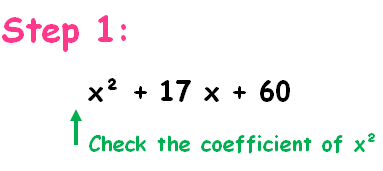Since it is 1. We are going to take the last number. That is 60 and we are going to factors of 60.

All terms are having positive sign. So we have to put positive sign for both factors.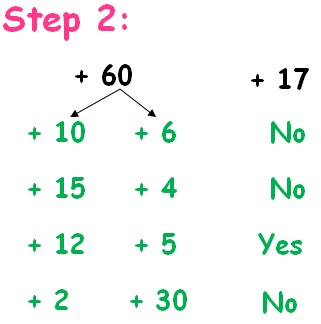Here,
10  x 6 = 60 but 10 + 6 = 16 not 17
15 x 4 = 60 but 15 + 4 = 19 not 17
12 x 5 = 60 and 12 + 5 = 17
2 x 30 = 60 but 2 + 30 = 32 not 17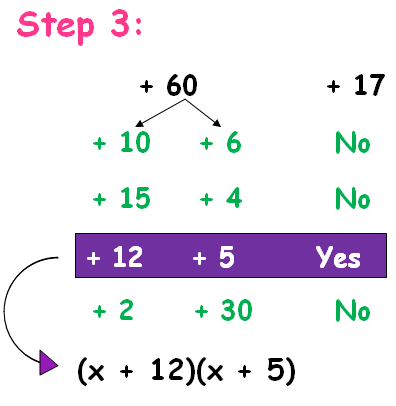(x + 12) (x + 5) are the factors of x² + 17 x + 60.

More examples of factoring quadratic equation
Example 8:
Factor 2 x² + x - 6
Solution :
To factor this quadratic equation we have to multiply the coefficient of x²  by the constant term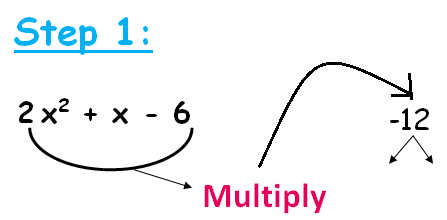So that we get -12, now we have to split -12 as the multiple of two numbers.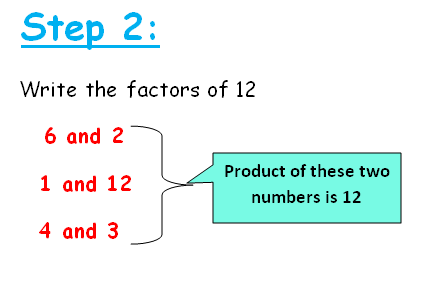Since the last term is having negative sign.So we have to put negative sign for the least number.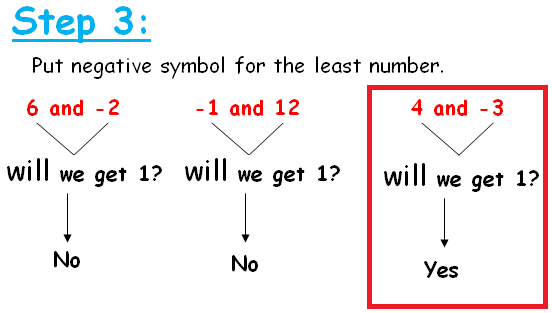Now we have to divide the two numbers 4 and -3 by the coefficient of x² that is 2.If it is possible we can simplify otherwise we have to write the numbers along with x.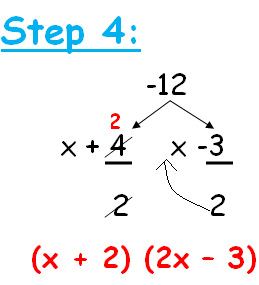So (x + 2) (2x - 3) are the factors of 2 x² + x - 6

Algebraic Identities
Factoring polynomials using algebraic identities (a+b)²and (a-b)²
Example 1:
Factorize 9x² - 24xy + 16y²
Solution:
Step 1:
To factor these kind of expressions first we try to write the first and last term as squares.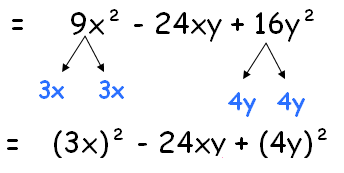Step 2:
Since it is possible,we have to split the middle term as shown the below picture.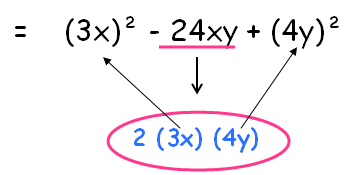We write the middle term 24xy as the multiple of 2 xfirst term x last term

Step 3:
Comparing these terms with the algebraic identity.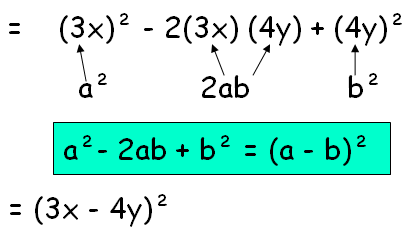(3x - 4y) (3x -4y) are the factors

Example 2:
Factorize 4x² + 12xy + 9y²
Solution:
Step 1:
To factor these kind of expressions first we try to write the first and last term as squares.
= 4x² + 12xy + 9y²
= 2² x² + 12 xy + 3²y²
= (2x)² + 12 xy + (3y)²

Step 2:
Since it is possible,we can to split the middle term as the multiple of 2 times product of first and last term
= (2x)² + 2 (2x) (3y) + (3y)²

Step 3:
Comparing these terms with the algebraic identity.
a² + 2ab + b² = (a + b)²
= (2x + 3y)²
(2x + 3y)(2x + 3y) are the factors

Example 3:
Factorize 16a² - 8a + 1
Solution:
Step 1:
To factor these kind of expressions first we try to write the first and last term as squares.
16a² - 8a + 1
= 4² a² - 8a + 1²
= (4a)² - 8a + (1)²

Step 2:
Since it is possible,we can to split the middle term as the multiple of 2 times product of first and last term
= (4a)² - 2 (4a) (1) + (1)²

Step 3:
Comparing these terms with the algebraic identity.
a² - 2ab + b² = (a - b)²
= (4a - 1)²

Factoring polynomials using algebraic identities a²- b²

Example 4:
Factorize 16a² - 9b²
Solution:
16a² - 9b²
= 4² a² - 3²b²
= (4a)² - (3b)²
The above algebraic expression exactly matches the identity a² - b²
Formula for a² - b² is (a + b) (a - b). In the above expression we have "4a" instead of "a" and "3b" instead of "b".
= (4a + 3b) (4a - 3b)

Example 5:
Factorize (a + b)² - (a - b)²
Solution:
Let x = a + b  and y = a - b
= x² - y²
The above algebraic expression exactly matches the identity a² - b²
Formula for a² - b² is (a + b) (a - b). In the above expression we have "x" instead of "a" and "y" instead of "b".
= (x + y) (x - y)
= [(a + b) + (a - b)] [(a + b) - (a -b)]
= (a + b + a - b) (a + b - a + b)
= 2a (2b)
= 4 a b

Factoring polynomials using algebraic identities a³+b³and a³-b³
Example 6:
Factorize 8x³ - 125y³
Solution:
Step 1:
Let us try to write the numbers 8 and 125 in term of cube.

8 = 2³ and 125 = 5³

= 2³ x³ - 5³y³

= (2x)³ - (5y)³

Step 2:
The above algebraic expression exactly matches the identity a³ - b³.
Formula for a³ - b³ is (a - b) (a² + ab + b²)
= (2x - 5y) [ (2x)² + (2x) (5y) + (5y)²]
= (2x - 5y) [ 4x² + 10xy + 25y²]

Example 7:
Factorize 27x³ + 64y³
Solution:
Step 1:
Let us try to write the numbers 27 and 64 in term of cube.
27 = 3³ and 64 = 4³
= 3³ x³ + 4³y³
= (3x)³ + (4y)³

Step 2:
The above algebraic expression exactly matches the identity a³ + b³.
Formula for a³ + b³ is (a + b) (a² - ab + b²)
= (3x + 4y) [ (3x)² - (3x) (4y) + (4y)²]
= (3x + 4y) [ 9x² - 12xy + 16y²]

Offer running on EduRev: Apply code STAYHOME200 to get INR 200 off on our premium plan EduRev Infinity!

## Mathematics (Maths) Class 9

190 videos|233 docs|82 tests

,

,

,

,

,

,

,

,

,

,

,

,

,

,

,

,

,

,

,

,

,

;